Students can download Maths Chapter 8 Statistics and Probability Ex 8.4 Questions and Answers, Notes, Samacheer Kalvi 10th Maths Guide Pdf helps you to revise the complete Tamilnadu State Board New Syllabus, helps students complete homework assignments and to score high marks in board exams.

## Tamilnadu Samacheer Kalvi 10th Maths Solutions Chapter 8 Statistics and Probability Ex 8.4

Question 1.
If P (A) = $$\frac{2}{3}$$, P(B) = $$\frac{2}{5}$$, P(A ∪ B) = $$\frac{1}{3}$$, then find P(A ∩ B).
P(A ∪ B) = P (A) + P (B) – P (A ∩ B)
$$\frac{1}{3}=\frac{2}{3}+\frac{2}{5}$$ – P (A ∩ B)Question 2.
A and B are two events such that, P(A) = 0.42, P(B) = 0.48, and P(A∩B)=016. Find (i) P(not A)
(ii) P(not B)
(iii) P(A or B)
Solution:
(a) P(A) = 0.42 ;
P(B) = 0.48
P(A∩B) = 0.16
(i) P(not A) = P($$\overline{\mathbf{A}}$$) = 1 – P(A) = 1 – 0.42 = 0.58
(ii) P(not B) = P($$\overline{\mathbf{B}}$$) = 1 – P(B) = 1 – 0.48 = 0.52
(iii) P(A or B) = P(A∪B) = P(A) + P(B) – P(A∩B)
= 0.42 + 0.48 – 0.16
= 0.74

Question 3.
If A and B are two mutually exclusive events of a random experiment and P (not A) = 0.45, P (A ∪ B) = 0.65, then find P(B).
P(not A) = 0.45
1 – P (A) = 0.45
P (A) = 1 – 0.45 = 0.55
P(A ∪ B) = P (A) + P (B)
0. 65 = 0.55 + P(B)
0. 65 – 0.55 = P(B)
0.10 = P (B)
P(B) = 0.1

Question 4.
The probability that atleast one of A and B occur is 0.6. If A and B occur simultaneously with probability 0.2, then find P($$\overline{\mathbf{A}}$$) + P($$\overline{\mathbf{B}}$$).
Solution:
P(A∪B) = 0.6
P(A∩B) = 0.2
P(A) + P(B) = [1 – P(A∪B)] + [1 – P(A∩B)] = [1 – 0.6] + [1 – 0.2]
= 0.4 + 0.8 = 1.2

Question 5.
The probability of happening of an event A is 0.5 and that of B is 0.3. If A and B are mutually exclusive events, then find the probability of neither A nor B happen.
Here P(A) = 0.5, P (B) = 0.3
P(A ∪ B) = P (A) + P(B) [A and B are mutually exclusive]
= 0.5 + 0.3
= 0.8
Probability of neither A nor [P(A ∪ B)’] = 1 – P(A ∪ B) = 1 – 0.8 = 0.2

Question 6.
Two dice are rolled once. Find the probability of getting an even number on the first die or a total of face sum 8.
Sample space = {(1, 1), (1, 2), (1, 3), (1, 4), (1, 5), (1, 6), (2, 1), (2, 2), (2, 3), (2, 4), (2, 5), (2, 6), (3, 1), (3, 2), (3, 3), (3, 4), (3, 5), (3, 6), (4, 1), (4, 2), (4, 3), (4, 4), (4, 5), (4, 6), (5, 1), (5, 2), (5, 3), (5, 4), (5, 5), (5, 6), (6, 1), (6, 2), (6, 3), (6, 4), (6, 5), (6, 6)}
n (S) = 36
Let A be the event of getting an even number on the first time
A = {(2, 1), (2, 2), (2, 3), (2, 4), (2, 5), (2, 6), (4, 1), (4, 2), (4, 3), (4, 4), (4, 5), (4, 6), (6, 1), (6, 2), (6, 3), (6, 4), (6, 5), (6, 6)}
n (A) = 18
$$P(A)=\frac{n(A)}{n(S)}=\frac{18}{36}$$
(ii) Let B be the event of getting a total of face sum 8.
B = {(2, 6) (3, 5) (4, 4) (5, 3) (6, 2)}
n(B) = 5
$$P(B)=\frac{n(B)}{n(S)}=\frac{5}{36}$$
A ∩ B = {(2, 6) (4, 4) (6, 2)}
n(A ∩ B) = 3
P(A ∩ B) = $$\frac{3}{36}$$
P(A ∪ B) = P (A) + P (B) – P (A ∩ B)The required probability = $$\frac{5}{9}$$

Question 7.
From a well-shuffled pack of 52 cards, a card is drawn at random. Find the probability of its being either a red king or a black queen.
n(S) = 52
Let A be the event of getting a red king
n(A) = 2
$$P(A)=\frac{n(A)}{n(S)}=\frac{2}{52}$$
Let B be the event of getting a black Queen king
n(B) = 2
$$P^{\prime}(B)=\frac{n(B)}{n(S)}=\frac{2}{52}$$
It A and B are mutually exclusive
P(A ∪ B) = P(A) + P(B)
$$=\frac{2}{52}+\frac{2}{52}=\frac{4}{52}=\frac{1}{13}$$
The required probability is $$\frac{1}{13}$$

Question 8.
A box contains cards numbered 3, 5, 7, 9,… 35, 37. A card is drawn at random from the box. Find the probability that the drawn card have either multiples of 7 or a prime number.
Sample space = {3, 5, 7, 9,…….,35, 37}
n(S) = 18
Let A be the event of getting a multiple of 7
A = {7, 21, 35}
n(A) = 3
$$P(A)=\frac{n(A)}{n(S)}=\frac{3}{18}$$
Let B be the event of getting a prime number
B = {3, 5, 7,11, 13, 17, 19, 23, 29, 31, 37}
n(B) = 11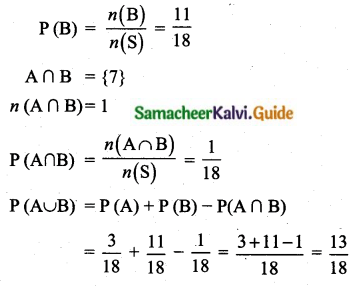Probability of getting a multiple of 7 or a prime number = $$\frac{13}{18}$$

Question 9.
Three unbiased coins are tossed once. Find the probability of getting atmost 2 tails or atleast 2 heads.
Sample space = {HHH, HHT, HTH, HTT, THH, THT, TTH, TTT}
n(S) = 8
Let A be the event of getting atmost 2 tails.
A = {HTT, THT, TTH, HHT, HTH, THH, HHH}
n(A) = 7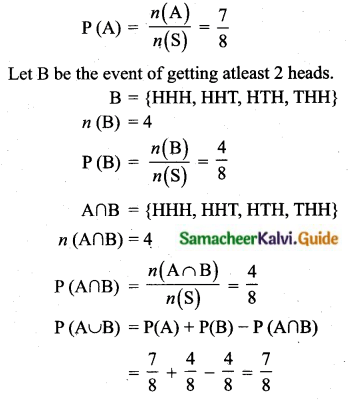Probability of getting atmost two tails or atleast 2 heads = $$\frac{7}{8}$$

Question 10.
The probability that a person will get an electrification contract is $$\frac{3}{5}$$ and the probability that he will not get plumbing contract is $$\frac{5}{8}$$. The probability of getting atleast one contract is $$\frac{5}{7}$$. What is the probability that he will get both?
Let A and B represent the event of getting electrification control and plumbing contract.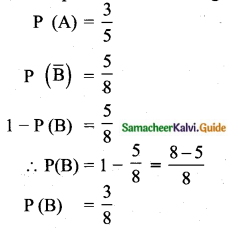Probability of getting both the job is $$\frac{73}{280}$$

Question 11.
In a town of 8000 people, 1300 are over 50 years and 3000 are females. It is known that 30% of the females are over 50 years. What is the probability that a chosen individual from the town is either a female or over 50 years?
Total number of people in a town is 8000.
n(S) = 8000
Total number of females = 3000
Let A be the event of getting number of females
n(A) = 3000
$$P(A)=\frac{n(A)}{n(S)}=\frac{3000}{8000}$$
Number of people over 50 years = 1300
Let B be the event of getting number of people over 50 years.
n(B) = 1300
$$P(B)=\frac{n(B)}{n(S)}=\frac{1300}{8000}$$
Given 30% of the females are over 50 years.Proability of getting either a female or over 50 years = $$\frac{17}{40}$$

Question 12.
A coin is tossed thrice. Find the probability of getting exactly two heads or atleast one tail or two consecutive heads.
Sample space = {HHH, HHT, HTH, HTT, THH, THT, TTH, TTT}
n(S) = 8
Let A be the event of getting exactly two heads.
A = {HHT, HTH, THH}
n(A) = 3
$$P(A)=\frac{n(A)}{n(S)}=\frac{3}{8}$$
Let B be the event of getting atleast one tail
B = {HHT, HTH, HTT, THH, THT, TTH, TTT}
n(B) = 7
$$P(B)=\frac{n(B)}{n(S)}=\frac{7}{8}$$
Let C be the event of getting consecutively
C = {HHH, HHT, THH}
n(C) = 3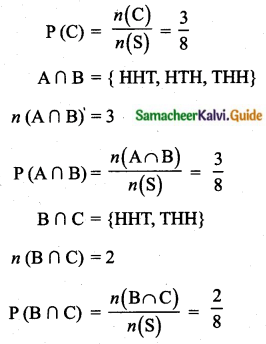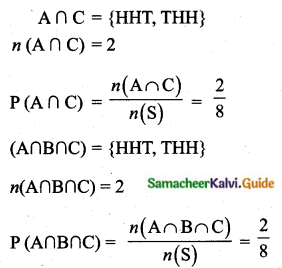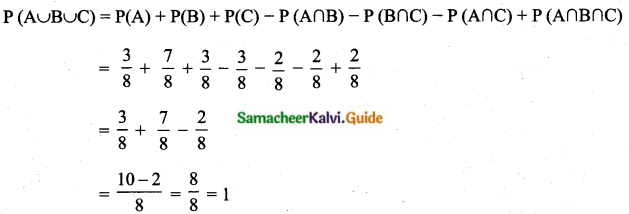The probability is 1.

Question 13.
If A, B, C are any three events such that probability of B is twice as that of probability of A and probability of C is thrice as that of probability of A and if P (A ∩ B) = $$\frac{1}{6}$$, P(B ∩ C) = $$\frac{1}{4}$$, P(A ∩ C) = $$\frac{1}{8}$$, P(A ∪ B ∪ C) = $$\frac{9}{10}$$ and P (A ∩ B ∩ C) = $$\frac{1}{15}$$, then find P(A), P(B) and P(C)?
By the given condition,
P(B) = 2 P(A), P(C) = 3 P(A)
P(A ∪ B ∪ C) = P(A) + P(B) + P(C) – P(A ∩ B) – P(B ∩ C) – P(A ∩ C) + P(A ∩ B ∩ C)Question 14.
In a class of 35, students are numbered from 1 to 35. The ratio of boys and girls is 4 : 3. The roll numbers of students begin with boys and end with girls. Find the probability that a student selected is either a boy with prime roll number or a girl with composite roll number or an even roll number.
Sample space (S) = {1, 2, 3,… ,35}
n(S) = 35
Total number of students = 35
Number of boys = $$\frac{4}{7}$$ × 35 = 20 [Boys Numbers = {1, 2, 3,…, 20}]
Number of girls = $$\frac{3}{7}$$ × 35 = 15 [Girls Numbers = { 21, 22,…, 35}]
Let A be the event of getting a boy role number with prime number
A = {2, 3, 5, 7, 11, 13, 17, 19}
n(A) = 8
P(A) = $$\frac{n(\mathrm{A})}{n(\mathrm{S})}$$ = $$\frac{8}{35}$$
Let B be the event of getting girls roll number with composite number.
B = {21, 22, 24, 25, 26, 27, 28, 30, 32, 33, 34, 35}
n(B) = 12
P(B) = $$\frac{n(\mathrm{B})}{n(\mathrm{S})}$$ = $$\frac{12}{35}$$
Let C be the event of getting an even roll number.
C = {2, 4, 6, 8, 10, 12, 14, 16, 18, 20, 22, 24, 26, 28, 30, 32, 34}
n(C) = 17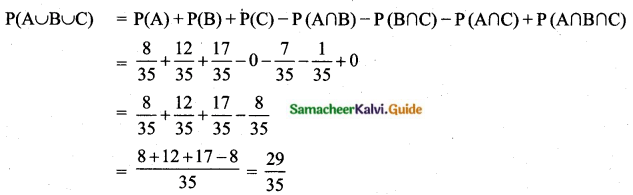Probability of getting roll number is $$\frac{29}{35}$$